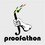# CMC - Problem 5

Problem 5. (6 points) Find the value of

$\sum_{m=1}^{8192}\left\lfloor\left\lfloor\frac{13}{1+\sum_{j=2}^m\left\lfloor\frac{(j-1)!+1}{j}-\left\lfloor\frac{(j-1)!}{j}\right\rfloor\right\rfloor}\right\rfloor^{1/13}\right\rfloor$Note by Cody Johnson
6 years, 7 months ago

This discussion board is a place to discuss our Daily Challenges and the math and science related to those challenges. Explanations are more than just a solution — they should explain the steps and thinking strategies that you used to obtain the solution. Comments should further the discussion of math and science.

When posting on Brilliant:

• Use the emojis to react to an explanation, whether you're congratulating a job well done , or just really confused .
• Ask specific questions about the challenge or the steps in somebody's explanation. Well-posed questions can add a lot to the discussion, but posting "I don't understand!" doesn't help anyone.
• Try to contribute something new to the discussion, whether it is an extension, generalization or other idea related to the challenge.

MarkdownAppears as
*italics* or _italics_ italics
**bold** or __bold__ bold
- bulleted- list
• bulleted
• list
1. numbered2. list
1. numbered
2. list
Note: you must add a full line of space before and after lists for them to show up correctly
paragraph 1paragraph 2

paragraph 1

paragraph 2

[example link](https://brilliant.org)example link
> This is a quote
This is a quote
    # I indented these lines
# 4 spaces, and now they show
# up as a code block.

print "hello world"
# I indented these lines
# 4 spaces, and now they show
# up as a code block.

print "hello world"
MathAppears as
Remember to wrap math in $$ ... $$ or $ ... $ to ensure proper formatting.
2 \times 3 $2 \times 3$
2^{34} $2^{34}$
a_{i-1} $a_{i-1}$
\frac{2}{3} $\frac{2}{3}$
\sqrt{2} $\sqrt{2}$
\sum_{i=1}^3 $\sum_{i=1}^3$
\sin \theta $\sin \theta$
\boxed{123} $\boxed{123}$

Sort by:

Suppose $k$ is prime, then it's quite easy to argue that $k \mid k!$ but $k \not \mid n!$ for any $n < k$, so $(k-1)! \equiv -1 \mod k$. In fact, it should be obvious that the other direction holds as well: $(k-1)! \equiv -1 \mod k \iff k \text{ is prime}$ Now, this suggests that only when $k$ is prime will $\frac{(k-1)!+1}{k}$ be an integer (since by definition $k$ divides $(k-1)! + 1$). Furthermore, since $0 < \frac{(k-1)!+1}{k} - \left\lfloor \frac{(j-1)!}{j} \right\rfloor \le 1$, then this will only be one iff $k$ is prime and zero otherwise: an indicator for primeness. In otherwords the summation on the denominator counts the number of primes up to $m$, aka the prime $\pi$ function.

From here it's easy sailing. Since the maximum of the expression $\frac{13}{1+\pi(m)}$ is 13, and because $1 < \sqrt{13} < 2$, each term will contribute exactly one to the sum until $\frac{13}{1+\pi(m)} < 1$. This will happen exactly when we hit the $13^{th}$ prime, which happens to be $41$, so the sum must have a value of $p_{13} - 1 = 40$.

- 6 years, 7 months ago

Correct! As your solution was posted before Michael Lee's, you get the 6 points. Great solution.

- 6 years, 7 months ago

Aww thank you so much, but I don't feel like it's fair to award the point to me since Michael solved the problem over an hour before I did, so I insist that the points should go to him! Thanks for the fun problems :)

- 6 years, 7 months ago

First complete solution, though.

- 6 years, 7 months ago

Note that the expression is undefined when $m = 1.$

- 6 years, 7 months ago

By standard math protocol, $\sum_{i=n+1}^ni=0$.

- 6 years, 7 months ago

Right!

- 6 years, 7 months ago

That's obvious....

- 6 years, 7 months ago

Note : $8192 = 2^{13}$

Suspicious.......

- 6 years, 7 months ago

I noticed that. -.-

- 6 years, 7 months ago

- 6 years, 7 months ago

YES! Solution?

- 6 years, 7 months ago

From where do you get such questions?

- 6 years, 7 months ago

Cody makes them.

- 6 years, 7 months ago

Wow, really nice! :)

- 6 years, 7 months ago

999?

- 6 years, 7 months ago

Proof: "The result will blow your mind. -- Cody Johnson"

- 6 years, 7 months ago

darn at first I thought that "CMC" meant "Canada Mathematics Competition".

- 6 years, 7 months ago